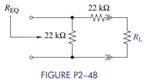Chat Now

# Select a value of R L in Figure P2−48 so that R EQ = 15 kΩ. Repeat for R EQ = 11 kΩ.

### Select a value of R L in Figure P2−48 so that R EQ = 15 kΩ. Repeat for R EQ = 11 kΩ.

Select a value of RL in Figure P2−48 so that REQ = 15 kΩ. Repeat for REQ = 11 kΩ.manish jayant 28-Jul-2020Get solution